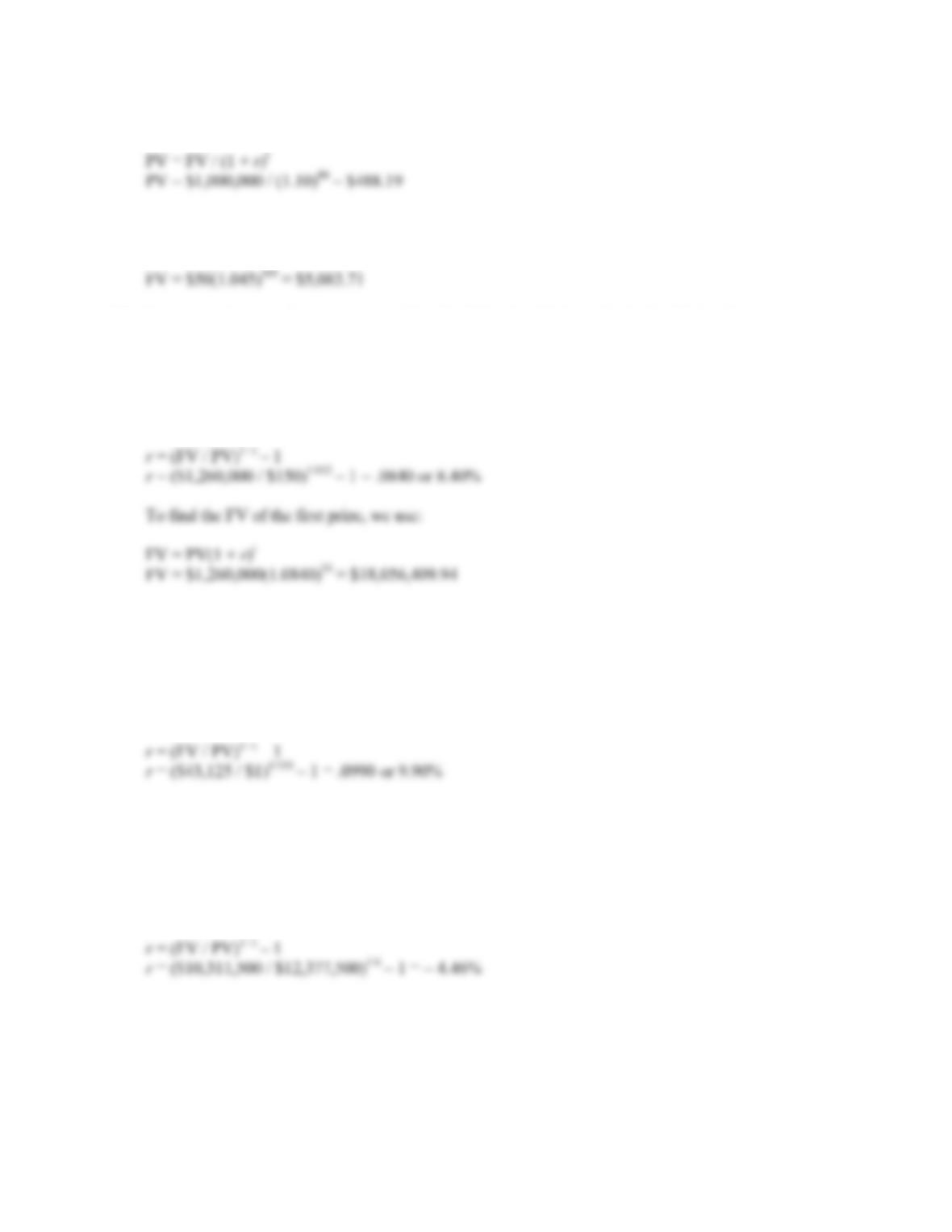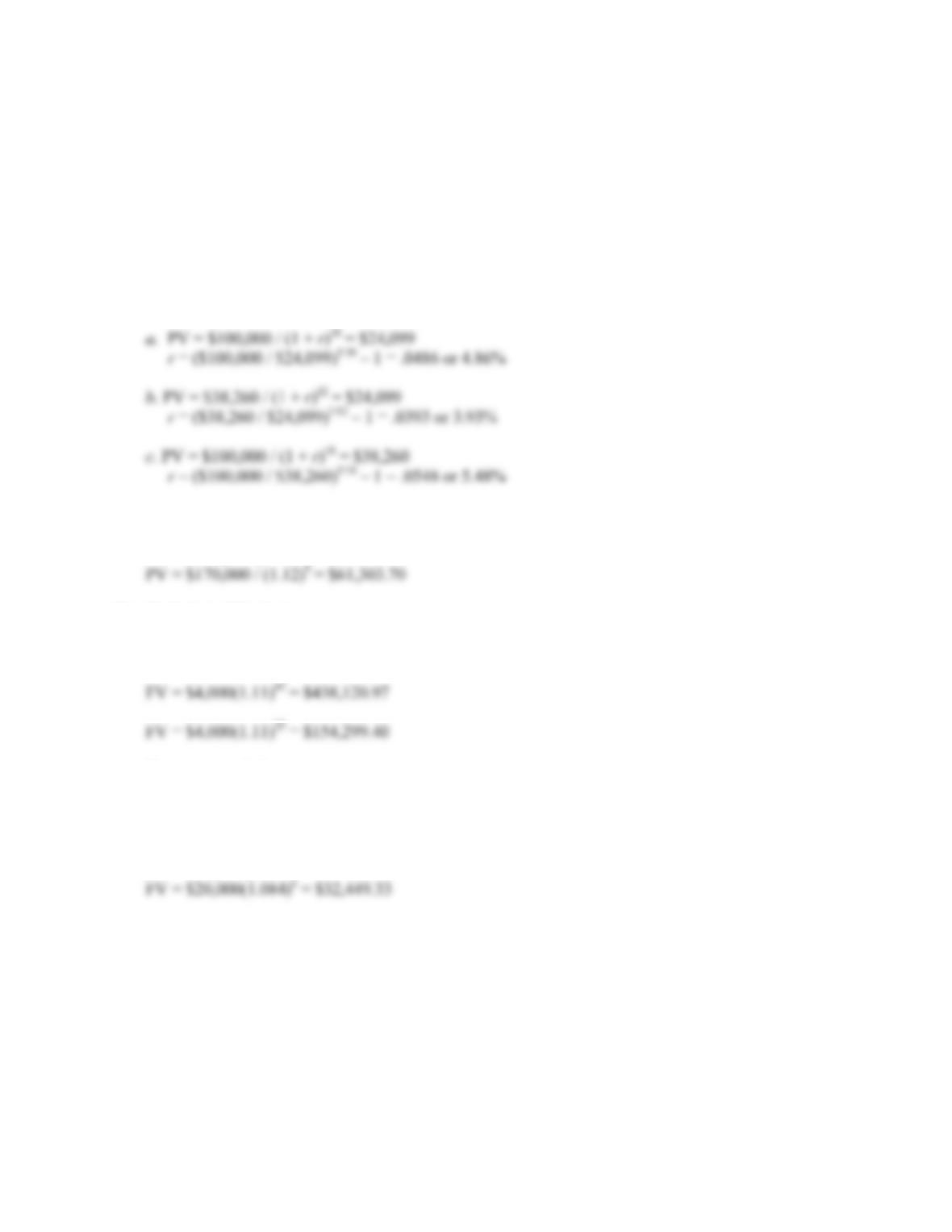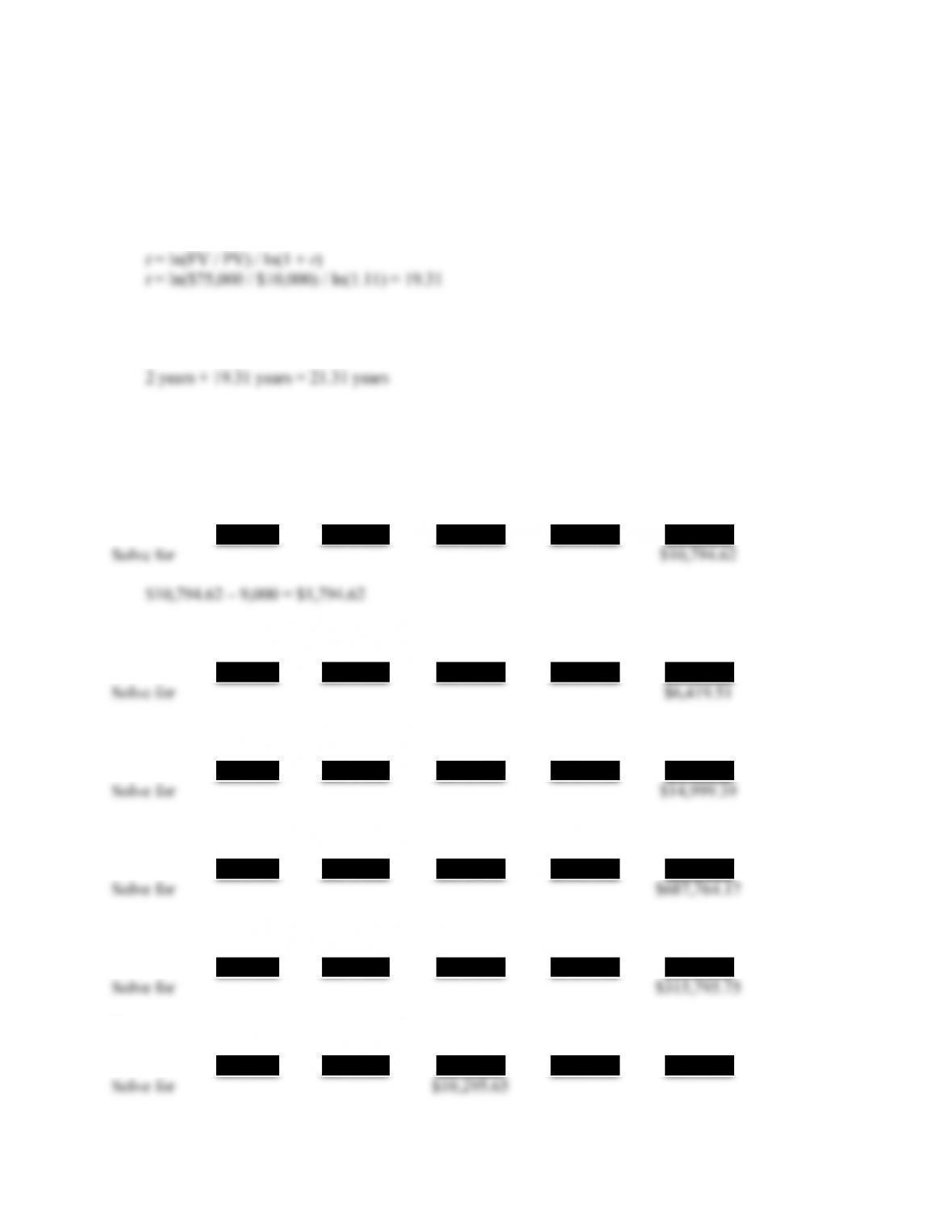# 978-0073382395 Chapter 5 Questions and Problems 11-20

Document Type
Homework Help
Book Title
Fundamentals of Corporate Finance Standard Edition 9th Edition
Authors
Stephen RossCHAPTER 5 B-61
11. To find the PV of a lump sum, we use:
12. To find the FV of a lump sum, we use:
FV = PV(1 + r)t
13. To answer this question, we can use either the FV or the PV formula. Both will give the same answer
since they are the inverse of each other. We will use the FV formula, that is:
FV = PV(1 + r)t
Solving for r, we get:
14. To answer this question, we can use either the FV or the PV formula. Both will give the same answer
since they are the inverse of each other. We will use the FV formula, that is:
FV = PV(1 + r)t
Solving for r, we get:
15. To answer this question, we can use either the FV or the PV formula. Both will give the same answer
since they are the inverse of each other. We will use the FV formula, that is:
FV = PV(1 + r)t
Solving for r, we get:
Notice that the interest rate is negative. This occurs when the FV is less than the PV.B-62 SOLUTIONS
Intermediate
16. To answer this question, we can use either the FV or the PV formula. Both will give the same answer
since they are the inverse of each other. We will use the FV formula, that is:
FV = PV(1 + r)t
Solving for r, we get:
r = (FV / PV)1 / t – 1
17. To find the PV of a lump sum, we use:
PV = FV / (1 + r)t
18. To find the FV of a lump sum, we use:
FV = PV(1 + r)t
Better start early!
19. We need to find the FV of a lump sum. However, the money will only be invested for six years, so
the number of periods is six.
FV = PV(1 + r)tCHAPTER 5 B-63
20. To answer this question, we can use either the FV or the PV formula. Both will give the same answer
since they are the inverse of each other. We will use the FV formula, that is:
FV = PV(1 + r)t
Solving for t, we get:
So, the money must be invested for 19.31 years. However, you will not receive the money for
another two years. From now, you’ll wait:
Calculator Solutions
1.
Enter 10 8% \$5,000
N I/Y PV PMT FV
2.
Enter 11 10% \$2,250
N I/Y PV PMT FV
Enter 7 8% \$8,752
N I/Y PV PMT FV
Enter 14 17% \$76,355
N I/Y PV PMT FV
Enter 8 7% \$183,796
N I/Y PV PMT FV
3.
Enter 6 7% \$15,451
N I/Y PV PMT FV

## Trusted by Thousands ofStudents

Here are what students say about us.